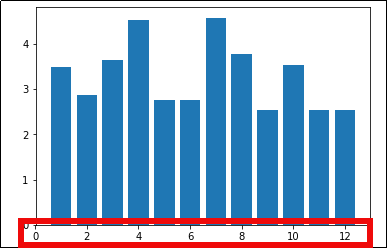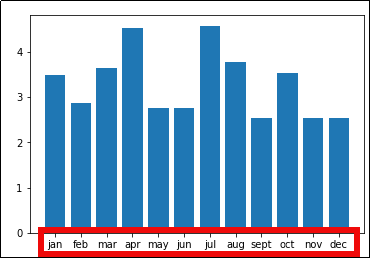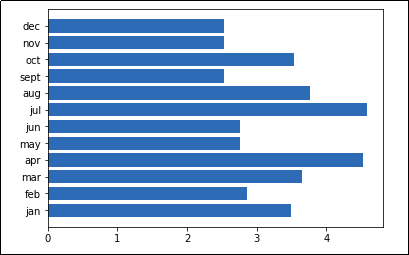Matplotlib Examples: Plots with String Axis Labels

Last updated:

WIP Alert This is a work in progress. Current information is correct but more content may be added in the future.

Bar plot

Full code available here

Just call plt.xticks(x_values, labels).

Example: use months of the year as the x-axis labels

import matplotlib.pyplot as plt
import numpy as np

# generate sample data for this example
xs = [1,2,3,4,5,6,7,8,9,10,11,12]
ys = np.random.normal(loc=3.0,size=12)
labels = ['jan','feb','mar','apr','may','jun','jul','aug','sept','oct','nov','dec']

plt.bar(xs,ys)

# HERE tell pyplot which labels correspond to which x values
plt.xticks(xs,labels)If you don't set labels,
each label is the value itselfSet string labels using plt.xticks(xs,labels)

Horizontal bar plot

Full code available here

Example: using the months of the year as the y-axis in a horizontal bar plot

import matplotlib.pyplot as plt
import numpy as np

# generate sample data for this example
xs = [1,2,3,4,5,6,7,8,9,10,11,12]
ys = np.random.normal(loc=3.0,size=12)
labels = ['jan','feb','mar','apr','may','jun','jul','aug','sept','oct','nov','dec']

plt.barh(xs,ys)

# HERE tell pyplot which labels correspond to which x values
plt.yticks(xs,labels)Horizontal bar plots are a useful alternative
when there is not enough space for labels
on the x (horizontal) axis

TODO